# Problem about dot product in probability density problem

• Another
The first line should be:\left( -i\nabla - \mathbf A \right)^2 \psi = \left( -i\nabla - \mathbf A \right) \cdot \left( -i\nabla - \mathbf A \right) \psiThe first line should be:\left( -i\nabla - \mathbf A \right)^2 \psi = \left( -i\nabla - \mathbf A \right) \cdot \left( -i\nabla - \math

#### Another

Homework Statement
probability density in magnetic field
Relevant Equations
## ∇ ⋅ (fg) = ∇f ⋅g + f(∇ ⋅ g) ##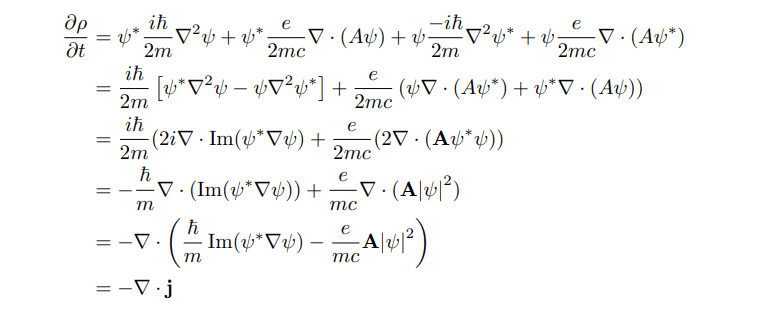I don't understand why ?
## \Psi ∇ ⋅ (A \Psi^ *) + \Psi ^* ∇ ⋅ (A \Psi ) = 2 ∇ ⋅(A \Psi ^* \Psi) ##
form
## ∇ ⋅ (fg) = ∇f ⋅ g + f(∇ ⋅ g) ##
Attempt at a Solution
## \Psi ∇ ⋅ (A \Psi^ *) + \Psi ^* ∇ ⋅ (A \Psi ) = 2 ∇ ⋅ (A \Psi ^* \Psi) - ∇\Psi ^* ⋅ A\Psi - ∇\Psi ⋅ (A\Psi ^*) ##

•Delta2
Another said:
Could you clarify the difference in meaning of "##A##" and "A" (going from lines 1 and 2, to line 3, in your attachment)? It looks like a scalar has changed to a vector.

Steve4Physics said:
Could you clarify the difference in meaning of "##A##" and "A" (going from lines 1 and 2, to line 3, in your attachment)? It looks like a scalar has changed to a vector.
this is full solution
http://www.physics.drexel.edu/~pgautam/wf/PHYS517/PHYS517HW1.pdf
You can see this problem in problem 2b in above link

I think ##A## is modulus of vector potential ##A = \sqrt(A^2)## and A is a vector quantities of vector potential vector A = A_x i + A_y j + A_zk

Another said:
this is full solution
http://www.physics.drexel.edu/~pgautam/wf/PHYS517/PHYS517HW1.pdf
You can see this problem in problem 2b in above link

I think ##A## is modulus of vector potential ##A = \sqrt(A^2)## and A is a vector quantities of vector potential vector A = A_x i + A_y j + A_zk

Going from line 2 to line 3 (in your Post #1 attachment) it appears that the scalar "##A##" (let’s assume it's the magnitude of A) magically changes to the vector A. If this is correct, it then raises the question: where did the additional information needed for A’s direction come from?

This makes no sense to me at all. My naturally cynical and suspicious nature makes me think it's an incorrect 'bodge' which accidentally works. But I'm no vector calculus expert and am more than happy to be corrected!

You might get more help if you posted in the “Advanced Physics Homework Help Forum” rather than here (the introductory forum).

•Delta2
If f and g are Scalars then:
##\nabla.(gf) ## has no meaning! One of them should be a vector. Further, in the attachment you provided in post #2, I found a lot of typos. Also, are you using any assumption for example: Coloumb Gauge(This problem can be solved without even assuming that gauge)?

•Delta2
Another said:
I don't understand why ?
## \Psi ∇ ⋅ (A \Psi^ *) + \Psi ^* ∇ ⋅ (A \Psi ) = 2 ∇ ⋅(A \Psi ^* \Psi) ##
This equation is not correct.

As @Abhishek11235 noted, there are several errors in the printed solutions. All of the ##A##'s should be boldface except for the terms with ##A^2##.

Here are the errors I found in the first few lines of the solutions: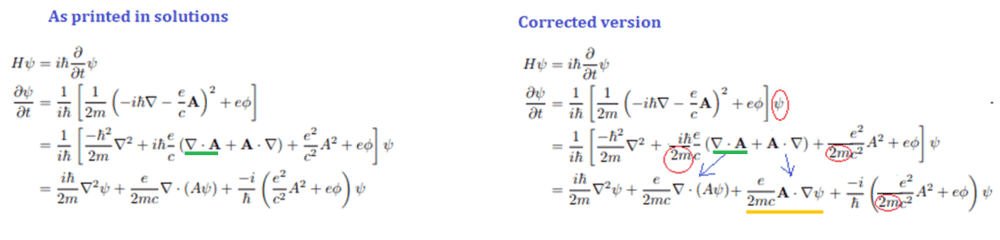In the next to last line, I've underlined a term in green. It's important to note that the divergence operator in this term acts on ##\mathbf A \psi## and not just on ##\mathbf A ##. So, you can see that the original solution is missing a term in the last line, which I underlined in yellow in the corrected version.

Similar corrections occur for the conjugated equation. With these corrections, you should be able to eventually get the desired result for ##\mathbf j##.

•JD_PM, PhDeezNutz, Steve4Physics and 2 others
@TSny i don't think we should add that term that is underlined in yellow. The term ##\nabla\cdot (\mathbf{A}\psi)## contains it because it is $$\nabla\cdot (\mathbf{A}\psi)=(\nabla\cdot \mathbf{A}) \psi+\mathbf{A}\cdot\nabla\psi$$ .

Delta2 said:
@TSny i don't think we should add that term that is underlined in yellow. The term ##\nabla\cdot (\mathbf{A}\psi)## contains it because it is $$\nabla\cdot (\mathbf{A}\psi)=(\nabla\cdot \mathbf{A}) \psi+\mathbf{A}\cdot\nabla\psi$$ .
Leaving out the messy constants, I find $$\left( -i\nabla - \mathbf A \right)^2 \psi = \left( -i\nabla - \mathbf A \right) \cdot \left( -i\nabla - \mathbf A \right) \psi = \left( -i\nabla - \mathbf A \right) \cdot \left( -i\nabla \psi -( \mathbf A \psi) \right)$$ $$= -\nabla^2 \psi + i\nabla \cdot (\mathbf A \psi)+ i \mathbf A \cdot \nabla \psi + A^2 \psi$$ $$= -\nabla^2 \psi + i(\nabla \cdot \mathbf A )\psi+2 i \mathbf A \cdot \nabla \psi + A^2 \psi$$

TSny said:
Leaving out the messy constants, I find $$\left( -i\nabla - \mathbf A \right)^2 \psi = \left( -i\nabla - \mathbf A \right) \cdot \left( -i\nabla - \mathbf A \right) \psi = \left( -i\nabla - \mathbf A \right) \cdot \left( -i\nabla \psi -( \mathbf A \psi) \right)$$ $$= -\nabla^2 \psi + i\nabla \cdot (\mathbf A \psi)+ i \mathbf A \cdot \nabla \psi + A^2 \psi$$ $$= -\nabla^2 \psi + i(\nabla \cdot \mathbf A )\psi+2 i \mathbf A \cdot \nabla \psi + A^2 \psi$$
I have my doubts about the first line, cause instead of calculating the square of the operator directly, you first apply the operator to the function ##\psi##, i just don't think your first equality is correct but i am not sure i might be wrong as well.

My result is close to the one mentioned in the OP but off by a factor of 2 maybe someone can spot my error (assuming the rest of my approach is right)

LaTeX plugin messed up so here's a picture of my work. Again I’m off by a factor of 2 somewhere.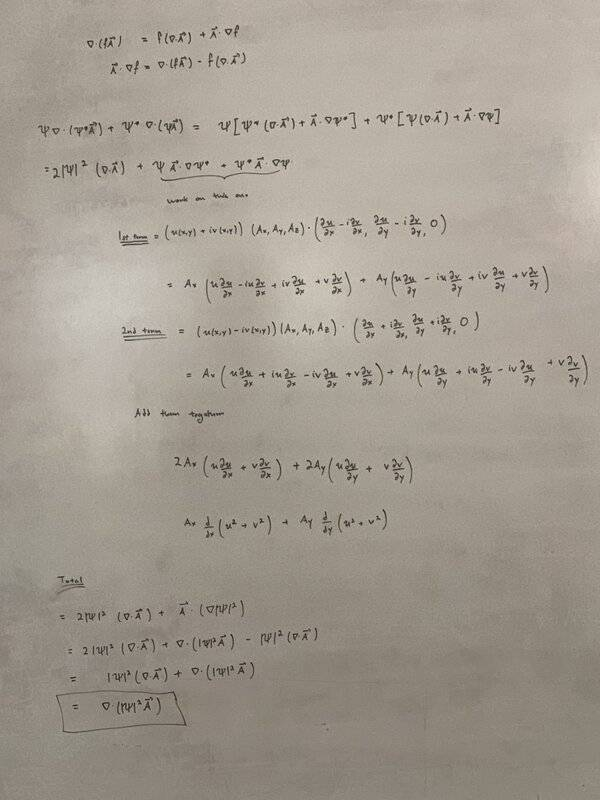If we keep the yellow-underlined term in post #6, we have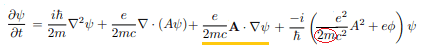We are interested in the middle two terms. Since they have a common factor of ##\frac{e}{2mc}##, I will leave out this factor for now. So, we want to consider $$\nabla \cdot (\mathbf A \psi) + \mathbf A \cdot \nabla \psi$$ which may be written as $$(\nabla \cdot \mathbf A) \psi+2\mathbf A \cdot \nabla \psi \,\,\,\,\,\,\,\, (1)$$

Similarly, the equation for ##\large \frac{\partial \psi^*}{\partial t}##, will yield $$(\nabla \cdot \mathbf A) \psi^*+2\mathbf A \cdot \nabla \psi^* \,\,\,\,\,\,\,\, (2)$$

Multiply (1) by ##\psi^*##, (2) by ##\psi##, and add $$\psi^* \left[( \nabla \cdot \mathbf A) \psi+2\mathbf A \cdot \nabla \psi \right] + \psi \left[( \nabla \cdot \mathbf A) \psi^*+2\mathbf A \cdot \nabla \psi^* \right]$$ $$=2(\nabla \cdot \mathbf A) |\psi|^2 + 2\psi^* \mathbf A \cdot \nabla \psi + 2\psi \mathbf A \cdot \nabla \psi^*$$ $$=2\left[ (\nabla \cdot \mathbf A) |\psi|^2 + \mathbf A \cdot \nabla(\psi^*\psi)\right] = 2\nabla \cdot (\mathbf A |\psi|^2)$$ Putting back the factor ##\frac{e}{2mc}##, gives $$\nabla \cdot \left ( \frac{e}{mc}\mathbf A |\psi|^2 \right)$$ This is what we want for one of the terms of ##\mathbf j##.

•Abhishek11235
PhDeezNutz said:
My result is close to the one mentioned in the OP but off by a factor of 2 maybe someone can spot my error (assuming the rest of my approach is right)
You obtained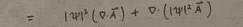This may be written as ##\,\,\, 2 \nabla \cdot (|\psi|^2 \mathbf A) - \mathbf A \cdot \nabla |\psi|^2##

The first term is what we want. The second term ends up being canceled by the yellow-underlined term in post #6 (and the corresponding term in the ##\psi^*## equation).

•PhDeezNutz
Delta2 said:
I have my doubts about the first line, cause instead of calculating the square of the operator directly, you first apply the operator to the function ##\psi##, i just don't think your first equality is correct but i am not sure i might be wrong as well.
What @TSny did is correct. In fact, it's a good way to do the calculation when you're not sure you're doing it correctly.

A similar situation comes up when you calculate the commutator of ##\hat x## and ##\hat p##. If you make the same mistake, you get this:
\begin{align*}
[\hat x,\hat p] &= x\left(\frac \hbar i \frac \partial {\partial x}\right) - \left(\frac \hbar i \frac \partial {\partial x}\right)x \\
&= -i\hbar \left( x \frac \partial {\partial x} - \frac\partial {\partial x}x \right) \\
&= -i \hbar \left( x \frac \partial {\partial x} - 1\right)
\end{align*} The error arises because the derivative in the second term doesn't act on just ##x## but on everything to the right of it, including the implied function the commutator acts on.

If you throw in a test function ##f##, then it's easy to see how it's supposed to work out:
\begin{align*}
[\hat x,\hat p]f &= x\left(\frac \hbar i \frac \partial {\partial x}\right)f - \left(\frac \hbar i \frac \partial {\partial x}\right)xf \\
&= -i\hbar \left( x \frac {\partial f} {\partial x} - \frac\partial {\partial x}xf \right) \\
&= -i\hbar \left( x \frac {\partial f} {\partial x} - f - x\frac {\partial f} {\partial x} \right) \\
&= i\hbar f
\end{align*}

•Delta2 and TSny